Excel Formula Sense Mel Babb

Let’s go over the basics of using formulas in Excel so you can get Excel to do more of the calculating work. After all, that’s one of the things Excel was designed to do excellently. By the way, most of what’s in this article also applies to other spreadsheet programs, like Microsoft Works and Quattro Pro. Excel is a spreadsheet program consisting of a grid of columns and rows in which among other things you enter numbers. Then you enter formulas and functions (special kinds of formulas) that perform mathematical calculations on the numbers you select and or enter in a formula.

How Excel Interprets a Formula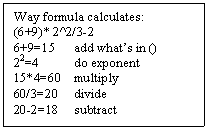Understanding how Excel processes information is absolutely necessary to getting the formula to produce the answer you want. When Excel runs a complicated calculation, it follows the basic algebra order of mathematical operations. It does not process the formula from left to right like we normally read. Instead, it processes in this order: 1) anything in parentheses (); 2) does exponents, ^ (e.g., a number raised to a power of 2, 3, etc. like 52); 3) multiplies and divides */; adds and subtracts +-. Look at Figure 1 to see how this calculates: (6+9)* 2^2/3-2.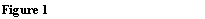So to make this perfectly clear: 3+5*2=13 and (3+5)*2=16. Following the rules, the first example multiplies first then adds, while the second example adds what is in the parentheses first, then multiplies. An exponent is indicated with the ^ caret sign between the number and the power, so 3^2 means 32, three squared. You can have more than one set of parentheses in a formula. When that happens, the inner most set of parentheses is done first.
Some Terminology
Creating formulas in Excel (or math for that matter) is rule bound. If you don’t create formulas often, the rules called syntax can seem as complex and convoluted as income tax instructions. In fact, the syntax is very logical. The information that goes into a formula or function is called an argument. The most common kind of arguments are numbers (called values), cell references (like A12, or G80), Range names (explained below) or text. The math symbols like +,-,*,/ in the formulas are called operators. A cell is described by a column letter and row number, G3, M100. A1 is the first cell in a sheet. A sheet consists of 255 columns and 65,536 rows. The first 26 columns are labeled A-Z, the next 26 AA-AZ, and so forth to IV which is column 255.
What’s the Big Deal About Formulas?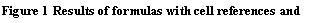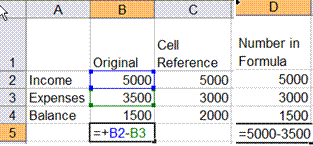Try These You’ll Like Them
Entering a formula is easier than describing it. Note that a formula can have a mixture of cell references and real numbers. Using the same example from figure 2, type in B6 =B4*2, to double the balance to 3000.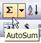Using a simple spreadsheet of expenses, let’s use the Sum function to total expenses and then see what happens if we increase expenses by 5, 10 or 15%. Once the raw numbers are in, and one formula is created, in this case a SUM function, that function can be copied to total the other expenses. Type the information in figure 3 into a spreadsheet. Click in cell B8. That’s where you want the total for Jan expenses. Click the AutoSum icon on the standard toolbar. Since adding up figures is one of the most common things done in spreadsheets, an icon exists to make that easy. Notice that a marquee appears around the numbers to be totaled. Click the icon again or just press enter and the column is totaled. To copy the formula to C8 and D8, use the Fill handle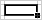point at the fill handle in the lower right corner of B8 until a black cross appears. Drag over cells C8 and D8. The formula is copied. Feb and Mar are now totaled. Click in E3, the total for year to date. Click the AutoSum icon, note the marquee, press enter. Copy the formula down using the fill handle.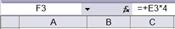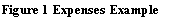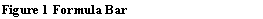Create a formula in F3 to project the expenses for a year. Type =, click E3, type*, type 4, press enter. Move the cursor to F3 The formula =E3*4 appears in the formula bar at the top of the screen and the answer in the cell.
There’s one more thing to cover about basic formula creation: the difference between relative and absolute cell reference. Sometimes when you copy a formula from one place to another, you want part of the formula to keep referring to exactly (absolutely) the same cell. In the expenses example this happens when you want to put a percentage increase into expenses. Part of the formula needs to move down a row as it is copied (move relative to where the answer is) and part of it needs to keep referring exactly (absolutely) to the same row and column. An indicator needs to be put in the part of the formula that stays constant. That indicator is a \$ (dollar sign), which is entered by pressing the F4 (function 4) key.
Try this example. In A11 type 10%, the percentage to increase expenses. In cell G3 type =, click F3, type (, click F3 again, press +, then ). Press *, Click A11, Press the function key F4, Press enter. Move the cursor back to G3. The formula bar displays =F3+(F3*\$A\$10). When you copy the formula down the F3 moves to F4 then F5, etc. while the A11 stays put. If you forget to press the F4 key, you can double click on G3 and click on the A11 side of the formula and press the F4 key to edit the formula. Then copy it down again. Try changing the percentage in A11 to 5% or 8% and watch the numbers in column G change. Now go ahead and see what kind of formulas you can create on your own.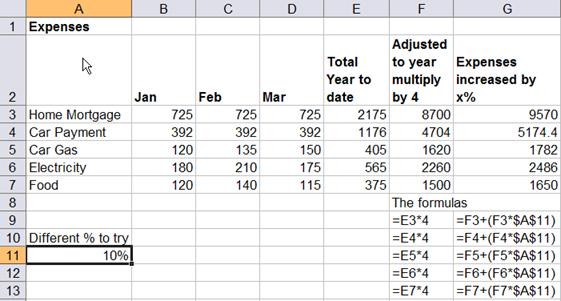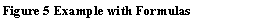Till next time. Happy Computing